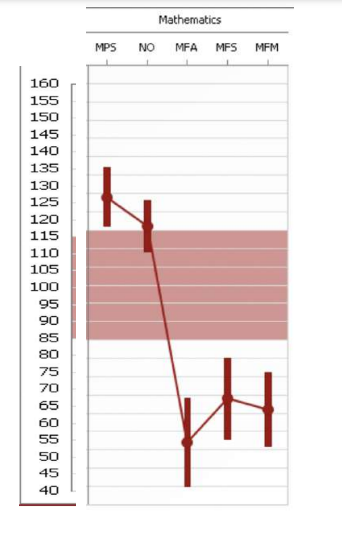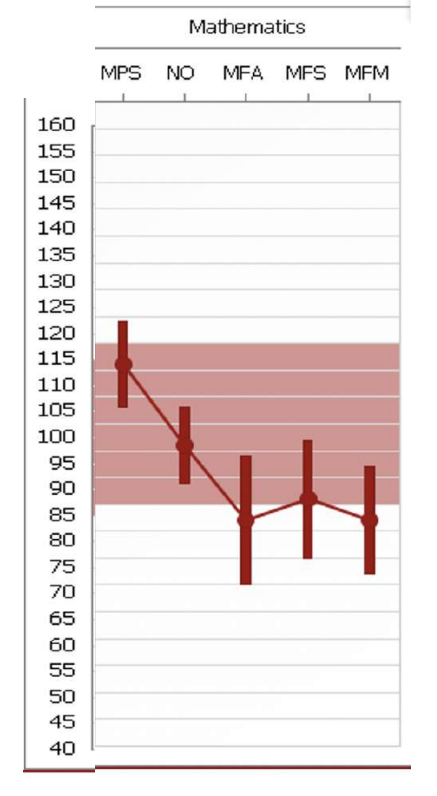#####How often do individuals with dyslexia also have dyscalculia, a specific disability in math?

As many as 60% of of dyslexic students may also be dyscalculic, but math difficulties are rarely tested for in schools, so specific needs for a particular student are rarely recognized or addressed.

It does not help that like dyslexia itself, dyscalculia can also present in different ways among dyslexic individuals.

##### DYSLEXICS USE DIFFERENT BRAIN AREAS WHILE PERFORMING MATH PROBLEMS

It was only in 2014 when neuroscientists found that dyslexic students doing simple math problems (addition and subtraction) us different brain areas to solve answers…in particular right parietal regions instead of left perisylvian areas which non-dyslexics use.

In our practice, when we performed comprehensive testing, we used The Wechsler Individual Achievement Test or WIAT to look at discrepancies that might exist between different abilities and achievements.

Although this high school student had a full scale IQ in the top 99th percentile, her basic math facts fluency (ability recall simple math facts – like 8+ 4) was at the 1st percentile, although her math problem solving ability was at the 93rd percentile.

The subtest scores at right abbreviations: MPS: Math Problem Solving, NO: Numerical Operations (untimed), MFA: Math Fluency Addition, MFS: Math Fluency Subtraction, MFM: Math Fluency Multiplication. The colored band refers to the “average” range.

Interestingly, Dr. Rachel Lambert of the University of California Santa Barbara has been researching differences in math among diverse individuals, including dyslexic mathematicians. What is so helpful about her approach is her interest in studying both strengths as well as challenges.

Strengths that dyslexic mathematicians noted related to visual thinking and a search for symmetries and mirror relationships, but for the focus of this article, another interesting aspect of her study, was how their career mathematicians had some difficulty with basic math facts fluency.

One mathematician said, “I couldn’t memorize facts in math. So I paid attention in class, and I had good enough teachers that they derived everything…” That statement was interesting for me to hear, because we had heard it so often before. Another mathematician recalled that their mother taught the multiplications tables through a smaller set of memorized facts – specifically the squares, then extrapolating to build other math relationships through the distributive property of known facts. Several of the mathematicians also noted the difficulty of retrieving math facts when they were also under time pressure.

Rachel’s conclusions:

“Offer opportunities for visual thinkers to learn new concepts through visual thinking. Not only provide multiple modalities for learning mathematics, but explicitly connect different kinds of representations. For example, one participant explained how their own mathematics teaching relies on visuals, but also with explicit connections to algebraic representations for those who preferred to think that way.

Remove the focus on memorization and procedural learning for students with dyslexia…

When mathematics focuses on speed and memorization “without structure,” the potential of those with dyslexia will not be realized in our schools.”

##### CALCULATOR ACCOMMODATIONS IN MATHIn the context of what is known about about neurobiological differences in math facts retrieval for dyslexic vs. non-dyslexic individuals, calculator accommodations should be a standard practice when the speed of math facts retrieval interferes with math problem solving.

Sometimes the discrepancies between math problem solving and basic math facts fluency is not as large as shown in the preceding example, but the discrepancy nevertheless was significant on a practical level that as a 3rd grader, his teachers were in discussion with his parents that he should be held back. His parents were exasperated at the situation because they thought their son was actually “good in math.”

With a nonverbal intelligence score at the 99th percentile and basic math facts fluency score at the 10th percentile, we successfully argued for calculator accommodations for this student and by the time this student was entering middle school, he had successfully skipped two grades in math with full calculator accommodations. Today he is graduating with a computer sciences degree and has won several engineering awards.

These cases are somewhat dramatic, but other research studies support wider use of calculators among dyslexic students.

Reviews of calculator use among students with “learning disabilities” or low math performing students showed that in general, calculators did not interfere with math learning, but in fact overall students’ improved math operational and problem solving skills.

From the paper:

“The operational skills of these students improved as a result of calculator use during instruction. Students received the most benefit when calculators had a pedagogical role in the classroom and were not just available for drill and practice or checking work. In order to have a positive influence on students’ operational skills, the findings suggest that calculator use during instruction should be long term (i.e., 9 or more weeks). With respect to problem solving, the skills of precollege students were not hindered by the inclusion of calculators in mathematics instruction. Based on a limited number of studies, the skills necessary to select the appropriate problem-solving strategies may improve as a result of calculator use…”

Research also showed that students’ attitudes about math improved (what a surprise!).

Because most dyslexic students are prone to having difficulty with automatization, math facts retrieval, and working memory overload, the use of calculators in regular use while learning new concepts and approaches to solving problems allows students to get farther in grasping the big picture of math concepts and algorithms.

Problems arise when basic math facts retrieval becomes the gatekeeper for higher math – the need even more urgent as schools feel pressure to have students make up for lost math learning because of the COVID pandemic.

##### FEWER PROBLEMS, MORE UNDERSTANDING, AND A CALCULATOR

Dyslexic students typically need to be assigned fewer homework problems, but be allowed to take as much time as they need to understand why certain practices of math problem solving make sense, and let them use a calculator for routine calculations (and graphing work in higher math) while they are working to understand why certain ways of solving a problem are correct.